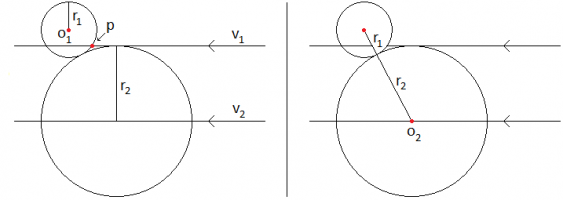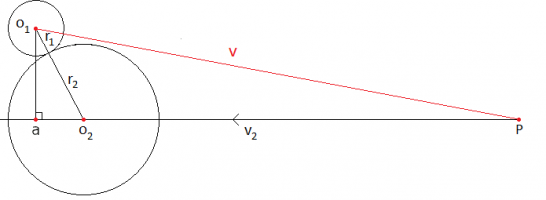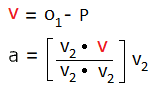# Position along vector for touching circles.

#### mook

##### New member
I'm coding a simple game with a friend, but I came across a problem I'm not even sure is solvable.
I've drawn a graphic to help explain what Information I have access to and what I need to determine.My problem is that I need to find the position of O2.

I know the 2 parallel vectors V1 and V2
I know the radius of both Circles r1 and r2
I know the cartesian location of O1
I know the cartesian location where the small circle first touches V1, that I've labelled P

I need to determine either the position O2 which is the large circle's origin along V2 where both circles touch. Or another vector through O1 -> O2 where a distance (r1 + r2) would take you to O2 from O1- though I guess finding one is basically finding the other.

Ultimately I need a function that would help me determine the position of O2 in any condition where r1 can change, and O1 is free to move anywhere it wants as long as it intersects V1 (making a P that's location will always be known)

If there is another piece of information necessary to generalise this problem I can see if its available, though I'm certain this is all the information I have access too.

I tried looking at some trivial cases but I just don't know my geometry, I hope I'm overthinking it and there's a trivial solution.

Thanks for having a look.

Last edited:

#### mook

##### New member
You're so right! I didn't even see the right angle triangle.....In this set-up there is also a parent ( P ) that projects the vectors - I didn't even consider P as useful so didn't include it in the OP...

After googling I found that with P , this new vector V can be used to project the point O1 onto V2 to determine aThen like you pointed out, using Pythagoras you can find the distance O2 -> a
Finally getting to O2 along V2 from either a or P

Thanks a lot!•Cubist